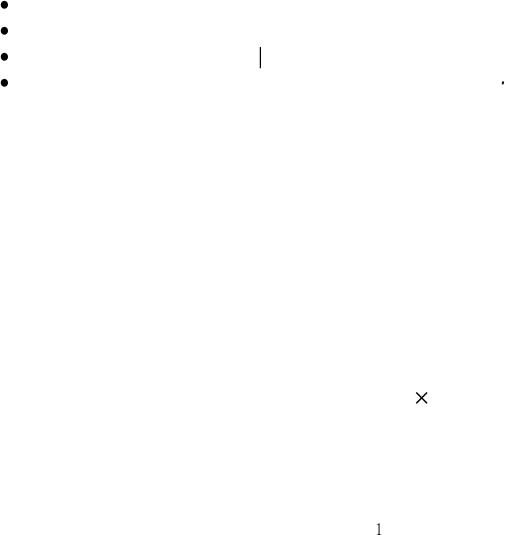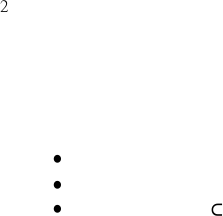Study Guides (390,000)
CA (150,000)
UTSG (10,000)
MAT (1,000)
Final

MAT237Y1 Final: Notes1Exam

Department
Mathematics
Course Code
MAT237Y1
Professor
John Bland
Study Guide
Final

This preview shows pages 1-2. to view the full 7 pages of the document.·
1
·
· | | | |
× × ×
2.1.
dot product and cross product.
Important points include:
Definitions of the dot product and cross product.
Cauchys Inequality and the Triangle Inequality.
The formula
a b
=
a b
cos
θ
.
if
u
is a unit vector and
v
is any vector, then (
u v
)
u
is the projection of
v
onto
the line generated by
u
.
This means that is we write
v
=
v
1
+
v
2
, where
v
1
is parallel to
u
and
v
2
is
orthogonal to
u
, then
v
1
=
(
u v
)
u
.
Equivalently, if we form a right triangle whose hypotenuse is
v
and whose base
lies along the line generated by
u
then the base is given by (
u
·
v
)
u
.
The meaning of the cross product:
a
×
b
is the vector that
is orthogonal to both
a
and
b
, with direction determined by the right-hand
rule, and
has length
|a| |b|
sin
θ
= area of the paralellogram generated by
a
and
b
.
algebraic properties of the cross product. For example
a × b
=
b × a
(
c
1
a
1
+
c
2
a
2)
b
=
c
1
a
1
b
+
c
2
a
2
b
etc.
The definition of the dot product, and all its properties mentioned above, should be familiar
from linear algebra.
Students should remember all of the above facts and should be able to use them to solve
problems.

Only pages 1-2 are available for preview. Some parts have been intentionally blurred.2
2.2.
open and closed sets. Students should know
the definition of a
sphere
and an (open)
ball
in
R
n
.
These are just matters of terminology and notation. We will normally say
ball
instead of (open) ball.
the definition of an
open
subset of
R
n
.
the definition of a
closed
subset of
R
n
.
Given a set
S
R
n
, you should know the definitions of its
interior Sint
boundary
∂S
,
closure
S
¯
.
the definition of a
bounded
subset of
R
n
.
A set is open if and only if its complement is closed.
You should also be able to use the definitions to solve problems at the level of the practice
problems. For example, you should be able to recognize open and closed sets and detemine
the interior/boundary/closure of a set.
Of the above concepts, the most important are probably
open
and
closed
. The textbook
gives two equivalent characterizations of open sets: a set
S
is
open
if it doesnt contain any
of its boundary points, or if every point of
S
is an interior point. We can also write
S
R
n
is
open
if, for every
x
S
, there exists
ε >
0 such that
B
(
ε,
x
)
S
.
This is just another way of saying that every point of
S
is an interior point.
We will later show that many sets can be instantly recognized as open or closed; see
Section 2.4.2 below.
Note that, contrary to what the names suggest, it is possible for a set to be both open
and closed at the same time.
2.2.1.
A remark.
Note that in his proof of Proposition 1.4, Folland asserts that
(1)
S
=
(
S
c
)
.
This follows directly by combining
the definition of a boundary point, and
the fact that (
S
c
)
c
=
S
If you are not convinced that (
S
c
)
c
=
S
or that (1) follows directly from combining from
the above considerations, then you should check these points in detail.
You're Reading a Preview

Unlock to view full version